# Running Machine Learning Applications on Docker

### My example of how to transfer a machine learning model to the living environment in the fastest and most effective way of using container infrastructures.

· AI Zone · Tutorial
Save
4.52K Views

As the projects about Machine Learning start to become widespread, there are more and more innovations about the practices related to how these projects are transferred to live environments. In this context, with this article, I will make an example of how to transfer a machine learning model to the living environment in the fastest and most effective way of using container infrastructures. I hope it will be a useful study in terms of awareness.

When we examine modern software development architectures today, we see that new practices and frameworks are developing in every step of the software development life cycle. We see that different approaches have been tried in every step from the development of the application to the testing and commissioning of the application. While examining modern software development architectures, we see that one of the most basic components we encounter is undoubtedly container technologies. Briefly, to mention the advantages that container technologies offer us;

• It enables the applications we develop to be run very easily and quickly.
• By keeping all the additional library dependencies required by the application integrated with our application, it enables us to deploy and distribute our application in an easy, fast, and effective.
• It is very easy to manage and maintain the applications put into use as containers.

Container technologies are an issue that needs to be examined in depth. Therefore, I will not go into more detail on this subject in this article. For more detailed information, you can review Docker documentation and read hundreds of blog articles about this subject.

In a world where we talk about container technologies, Docker is undoubtedly the locomotive technology. Docker, which has become a defacto standard in the industry, is a tool used by almost everyone who develops container-based software. Today, I will perform this example using Docker.

To make this example, Docker must be installed in the environment in which we work. You can prepare Docker in your working environment by following the line below.

First of all, let me talk a little bit about my script. I want to develop a sentiment analysis model. I will train this model with a data set collected from IMDB, yelp, and amazon and develop a simple sentiment prediction model. After finishing this model, I will design a web page and make the model available to end-users through this web page. Then, I will convert this application as a container image and run on Docker.

## Python   x 21 102 1 `import pandas as pd` 2 `import numpy as np` 3 `import pickle` 4 `import sys` 5 `import os` 6 `import io` 7 `import re` 8 `from sys import path` 9 `import numpy as np` 10 `import pickle` 11 `from sklearn.feature_extraction.text import CountVectorizer` 12 `from sklearn.naive_bayes import MultinomialNB` 13 `from sklearn.model_selection import train_test_split` 14 `from sklearn.preprocessing import LabelBinarizer` 15 `import matplotlib.pyplot as plt` 16 `from string import punctuation, digits` 17 `from IPython.core.display import display, HTML` 18 `from nltk.corpus import stopwords` 19 `from nltk.corpus import stopwords` 20 `from nltk.tokenize import word_tokenize` 21 `from nltk.tokenize import RegexpTokenizer` 22 23 24 `#Amazon Data` 25 `input_file = "../data/amazon_cells_labelled.txt"` 26 `amazon = pd.read_csv(input_file,delimiter='\t',header=None)` 27 `amazon.columns = ['Sentence','Class']` 28 29 `#Yelp Data` 30 `input_file = "../data/yelp_labelled.txt"` 31 `yelp = pd.read_csv(input_file,delimiter='\t',header=None)` 32 `yelp.columns = ['Sentence','Class']` 33 34 `#Imdb Data` 35 `input_file = "../data/imdb_labelled.txt"` 36 `imdb = pd.read_csv(input_file,delimiter='\t',header=None)` 37 `imdb.columns = ['Sentence','Class']` 38 39 40 `#combine all data sets` 41 `data = pd.DataFrame()` 42 `data = pd.concat([amazon, yelp, imdb])` 43 `data['index'] = data.index` 44 45 46 `#Total Count of Each Category` 47 `pd.set_option('display.width', 4000)` 48 `pd.set_option('display.max_rows', 1000)` 49 `distOfDetails = data.groupby(by='Class', as_index=False).agg({'index': pd.Series.nunique}).sort_values(by='index', ascending=False)` 50 `distOfDetails.columns =['Class', 'COUNT']` 51 `print(distOfDetails)` 52 53 `#Distribution of All Categories` 54 `plt.pie(distOfDetails['COUNT'],autopct='%1.0f%%',shadow=True, startangle=360)` 55 `plt.show()` 56 57 58 `#Text Preprocessing` 59 `columns = ['index','Class', 'Sentence']` 60 `df_ = pd.DataFrame(columns=columns)` 61 62 `#lower string` 63 `data['Sentence'] = data['Sentence'].str.lower()` 64 65 `#remove email adress` 66 `data['Sentence'] = data['Sentence'].replace('[a-zA-Z0-9-_.]+@[a-zA-Z0-9-_.]+', '', regex=True)` 67 68 `#remove IP address` 69 `data['Sentence'] = data['Sentence'].replace('((25[0-5]|2[0-4][0-9]|?[0-9][0-9]?)(\.|\$)){4}', '', regex=True)` 70 71 `#remove punctaitions and special chracters` 72 `data['Sentence'] = data['Sentence'].str.replace('[^\w\s]','')` 73 74 `#remove numbers` 75 `data['Sentence'] = data['Sentence'].replace('\d', '', regex=True)` 76 77 `#remove stop words` 78 `for index, row in data.iterrows():` 79 `    word_tokens = word_tokenize(row['Sentence'])` 80 `    filtered_sentence = [w for w in word_tokens if not w in stopwords.words('english')]` 81 `    df_ = df_.append({"index": row['index'], "Class":  row['Class'],"Sentence": " ".join(filtered_sentence[0:])}, ignore_index=True)` 82 83 `data = df_` 84 85 86 `from sklearn.metrics import confusion_matrix` 87 `from sklearn.model_selection import cross_val_score` 88 `from sklearn.metrics import accuracy_score` 89 `from sklearn.neural_network import MLPClassifier` 90 `from sklearn.feature_extraction.text import TfidfVectorizer` 91 `from sklearn.linear_model import SGDClassifier` 92 93 94 `#grid search result` 95 `vectorizer = TfidfVectorizer(analyzer='word',ngram_range=(1,2), max_features=50000,max_df=0.5,use_idf=True, norm='l2') ` 96 `counts = vectorizer.fit_transform(X_train)` 97 `vocab = vectorizer.vocabulary_` 98 `classifier = SGDClassifier(alpha=1e-05,max_iter=50,penalty='elasticnet')` 99 `targets = y_train` 100 `classifier = classifier.fit(counts, targets)` 101 `example_counts = vectorizer.transform(X_test)` 102 `predictions = classifier.predict(example_counts)` 103 104 `from sklearn.metrics import precision_score` 105 `from sklearn.metrics import recall_score` 106 `from sklearn.metrics import classification_report` 107 108 `#Model Evaluation` 109 `acc = accuracy_score(y_test, predictions, normalize=True)` 110 `hit = precision_score(y_test, predictions, average=None,labels=classes)` 111 `capture = recall_score(y_test, predictions, average=None,labels=classes)` 112 113 `print('Model Accuracy:%.2f'%acc)` 114 `print(classification_report(y_test, predictions))` 20 `from nltk.tokenize import word_tokenize`

Yes, we created our model. What we need next is to record the model we created and the vocabulary output of the model with the pickle. We will use these outputs on the web page we will develop later.

Python

`xxxxxxxxxx`
1

1
`### model_save ###`
2
`pickle.dump(classifier,open("model_sentiment.pkl","wb"))`
3
`pickle.dump(vocab,open("vocab_sentiment.pkl","wb"))`

Our outputs related to our model were recorded on a disk. Now we can start the development of the web page that will find the sentiment of the text that will be sent as input to this model and return.I will use python's flask library because I will do the development with python. A library that allows you to develop web-based applications with Flask python. I will develop a web service using this library. First of all, let's install the flask in our development environment.

Shell

`xxxxxxxxxx`
1

1
`pip install -U Flask`
2

3
`#OR`
4

5
`conda install -c anaconda flask`

Now that we have completed our Flask installation, we can now develop our website.

Before I start developing, I want to show the directory structure I have installed in my local. I think it would be useful for you to set up a similar directory structure for such a study. Since this will already be a web application, webform.py, which we write the main code, will look for the .html file that it will render while running, under the / templates directory. So after developing your HTML page, you will have to put it under the templates directory. Below you can see the directory structure that will work successfully.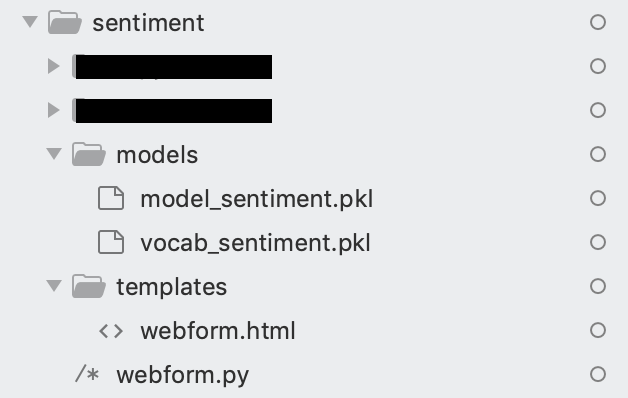As you can see in the directory production, I moved the model outputs that I developed and output to under the models folder. I created and put my webform.html file under the Templates directory. Likewise, I created and saved my code file named webform.py under the main directory.

Since this application will be delivered to the user via the web page, I design a simple HTML form where the user will enter text and see the result with a very simple design. This web page can be equipped with all the current infrastructures provided by web technologies, I just want to show how it is made with a simple design as much as possible.

### HTML   `xxxxxxxxxx` 1 1 `<form method="POST">` 2 ` <br><b>Enter Tweet here...</b></br>` 3 ` <textarea rows="4" cols="50" name="tweet">` 4 ` </textarea>` 5 ` <br></br>` 6 `    <input type="submit">` 7 `    <br></br>` 8 `    <h1><b> Result: </b> {{ value }} </h1>` 9 `</form>`

Now let's see what the form we created is like.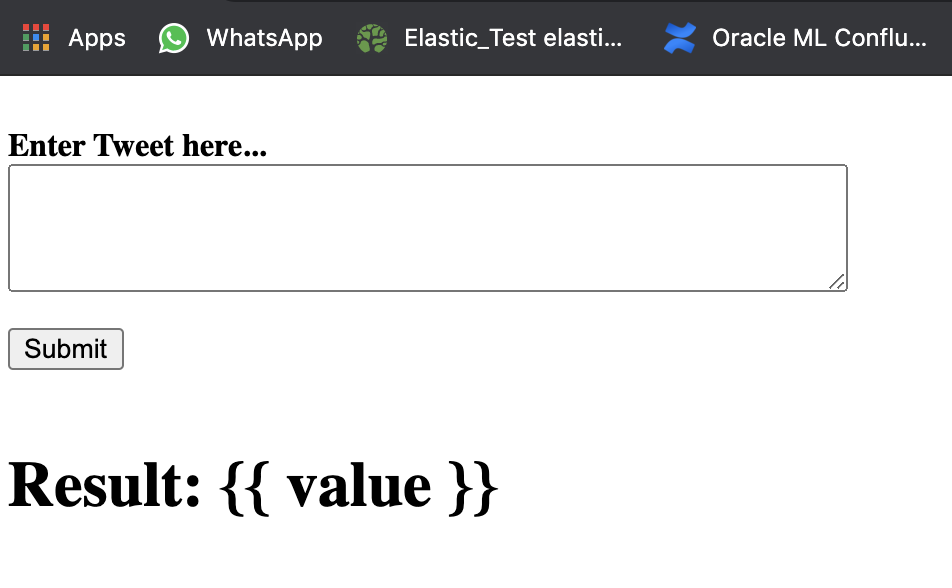Yes, as we can see, our design is very simple. Let's write your python code that will use this form and run our model, find the sentence of the text sent from the page above, and return the answer to the form.

## webform.py

There are four things to note here. The first one is how I called the HTML code I wrote. The second is to load our model outputs and make them ready for use.

The third one is to include the text that will come from outside as a parameter in a variable and the fourth one is returned to the web page.

Python

`xxxxxxxxxx`
1
61

1
`from flask import Flask, request, render_template, url_for`
2
`import pickle`
3
`import time`
4
`import os`
5
`from sklearn.feature_extraction.text import TfidfVectorizer`
6
`import pandas as pd`
7
`import io`
8
`import re`
9
`from sys import path`
10
`import numpy as np`
11
`from sklearn.feature_extraction.text import CountVectorizer`
12
`from sklearn.naive_bayes import MultinomialNB`
13
`from sklearn.model_selection import train_test_split`
14
`from sklearn.preprocessing import LabelBinarizer`
15
`import matplotlib.pyplot as plt`
16
`from string import punctuation, digits`
17
`from flask import Flask, request, render_template, url_for`
18

19
`start_time = time.time()`
20

21
`app = Flask(__name__)`
22
`app.config["DEBUG"] = True`
23

24
`@app.route('/')`
25
`def my_form():`
26
`    return render_template('webform.html')`
27

28
`@app.route('/', methods=['POST','GET'])`
29
`def home():`
30
`    vec = open("models/model_sentiment.pkl", 'rb')`
31
`    loaded_model = pickle.load(vec)`
32

33
`    vcb = open("models/vocab_sentiment.pkl", 'rb')`
34
`    loaded_vocab = pickle.load(vcb)`
35

36
`    txt = request.form['tweet']`
37
`    `
38
`    examples = txt`
39

40
`    examples = examples.lower()`
41
`    examples = examples.replace('\n',' ')`
42
`    examples = re.sub(r'[a-zA-Z0-9-_.]+@[a-zA-Z0-9-_.]+', ' ', examples)`
43
`    examples = re.sub(r'@[A-Za-z0-9]+', ' ', examples)`
44
`    examples = re.sub(r'((25[0-5]|2[0-4][0-9]|?[0-9][0-9]?)(\.|\$)){4}', ' ', examples)`
45
`    examples = re.sub(r'[^\w\s]', ' ', examples)`
46
`    examples = re.sub(r'\d', ' ', examples)`
47
`    examples = re.sub(' +',' ',examples) `
48
`    examples = [examples]`
49
`    `
50
`        from sklearn.feature_extraction.text import TfidfTransformer`
51
`    from sklearn.feature_extraction.text import TfidfVectorizer`
52
`    count_vect = TfidfVectorizer(analyzer='word',ngram_range=(1,2), max_features=50000,max_df=0.6,use_idf=True, norm='l2',vocabulary=loaded_vocab)`
53
`    x_count = count_vect.fit_transform(examples)`
54
`    predicted = loaded_model.predict(x_count)`
55
`    result=''`
56
`    if predicted == 0:`
57
`        result= 'Negative'`
58
`    elif predicted == 1:`
59
`        result= 'Positive'`
60
`    return render_template('webform.html',value=result)`

Yes, we have completed the developments. Let's run the webform.py code we wrote.

Shell

`xxxxxxxxxx`
1

1
`env FLASK_APP=webform.py flask run`

Yes, our service got up and the endpoint address was shown to us on the screen. Let's test the service. For the test, it will be enough to write the endpoints on the screen and we will do the test with an input we want.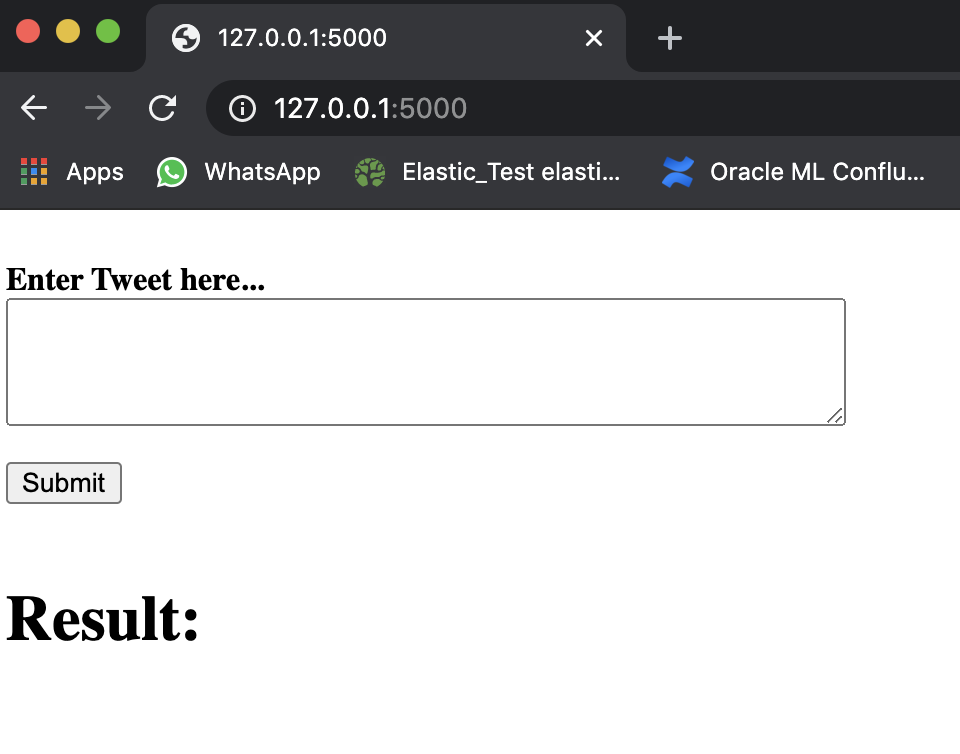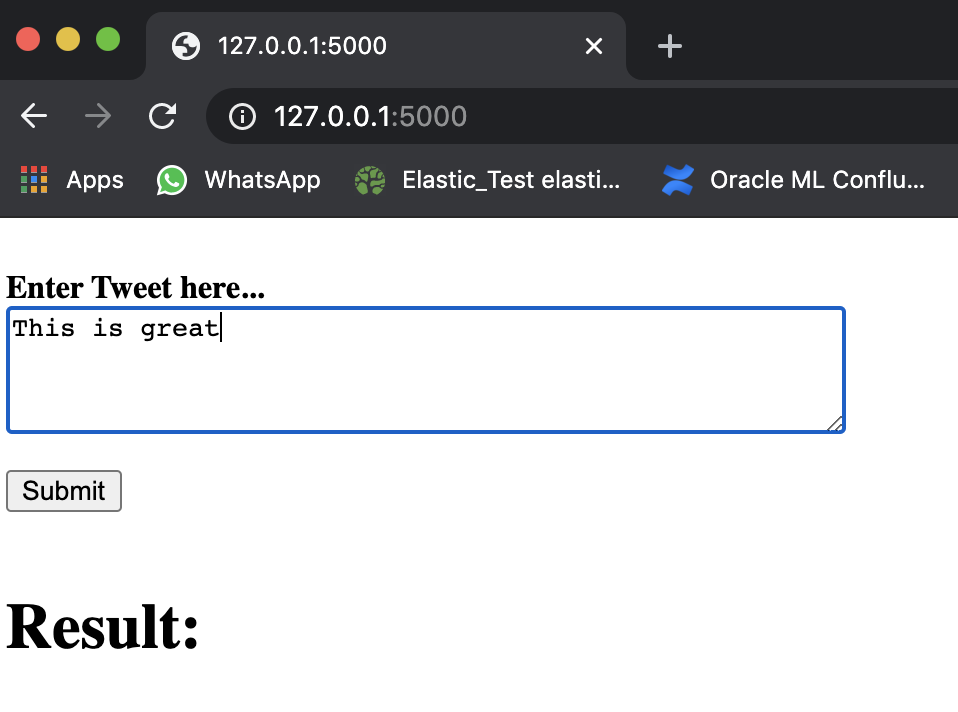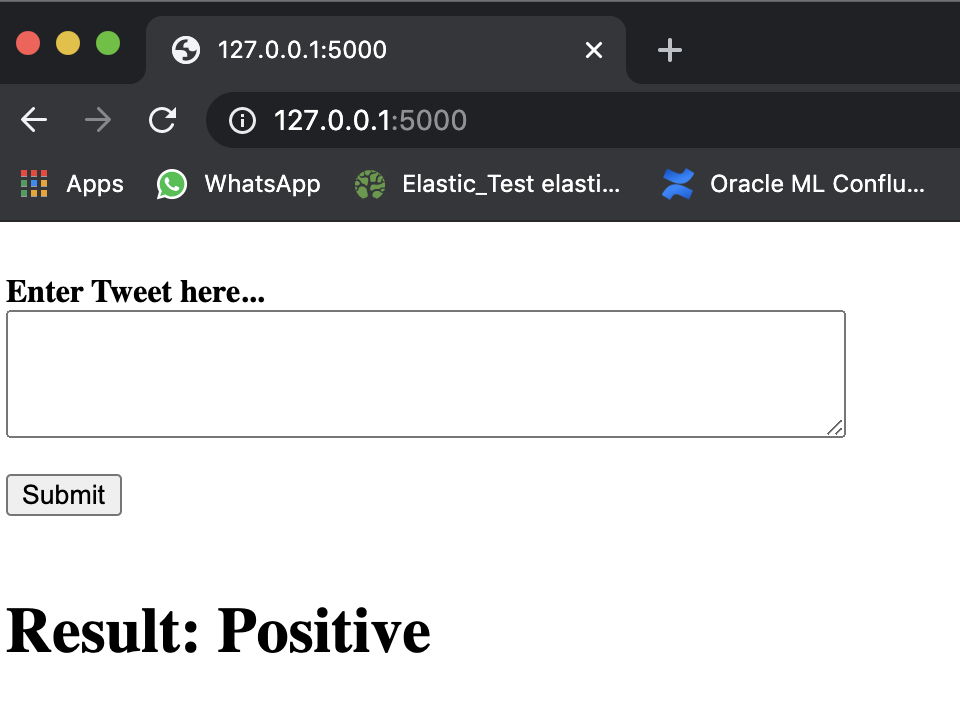Now let's write dockerfile to create my image that will turn this app into a Docker container. I will use the skeleton of the image I will create from the official python images in dockerhub.

## Dockerfile.yaml

YAML

`xxxxxxxxxx`
1
11

1
`FROM python:3.9.0b3-alpine3.12`
2
`WORKDIR /app`
3
`ENV FLASK_APP webform.py`
4
`ENV FLASK_RUN_HOST 0.0.0.0`
5
`RUN apk --update add gcc build-base freetype-dev libpng-dev openblas-dev`
6
`RUN apk --no-cache add --virtual .builddeps g++ musl-dev`
7
`RUN apk del .builddeps`
8
`RUN pip install scikit-learn==0.19.1`
9
`RUN pip install --no-cache-dir flask sklearn matplotlib pandas numpy `
10
`COPY . .`
11
`CMD ["flask", "run"]`

Now, let's run our code that will produce our image from Dockerfile we wrote on the terminal screen.

Shell

`xxxxxxxxxx`
1

1
`docker build -f Dockerfile.yaml -t sentiment-model .`

The image may take a long time to build. After the build process is finished, you can check whether the image comes to the Docker registry with the following command.

Shell

`xxxxxxxxxx`
1

1
`docker image ls`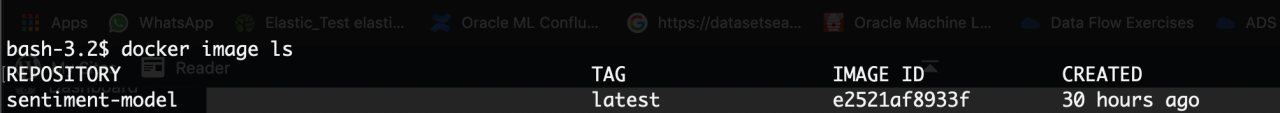Yes, as we have seen, our docker image has been created with the tag name we have provided.

Let's put this image in a container, run it, and test it.

Shell

`xxxxxxxxxx`
1

1
`docker run -d -it --rm -p 3000:5000 --name sentiment-model sentiment-model:latest`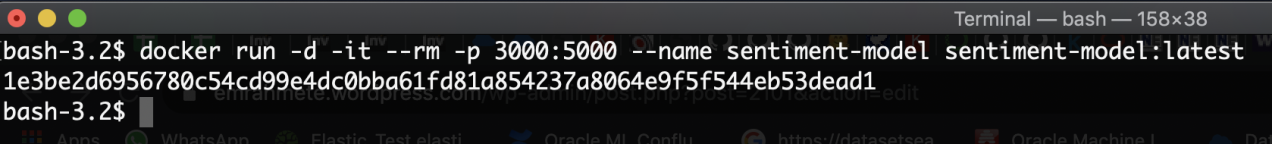Yes, the container stood up. Now let's try to access our application running in the container in our main machine.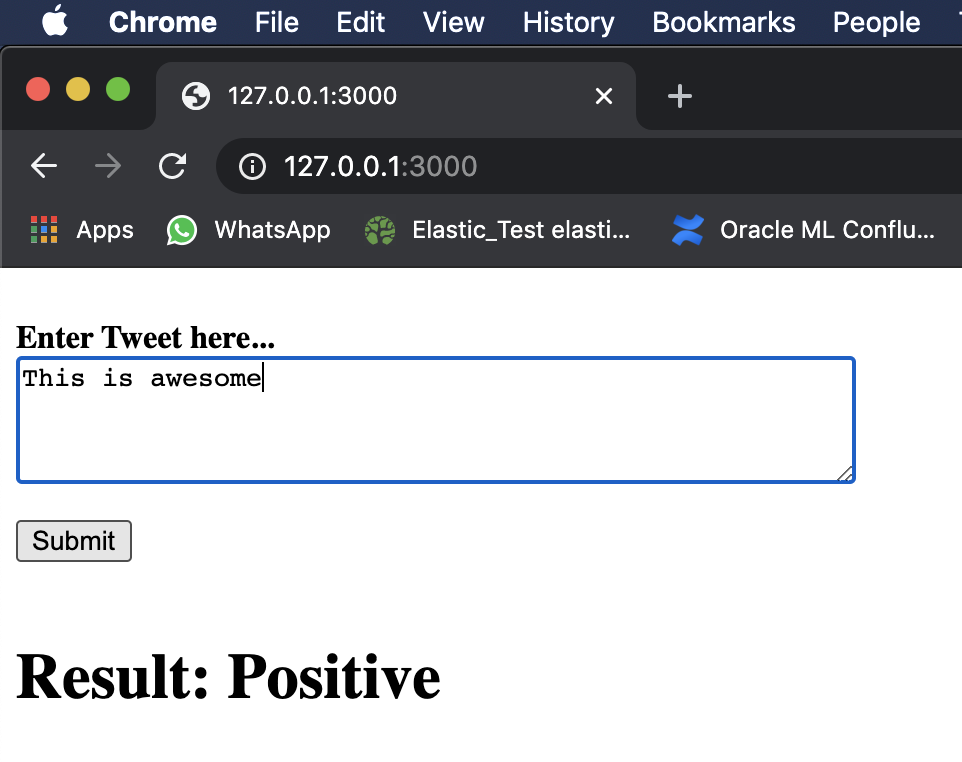Yes, we were able to reach the application we run through the container from our browser and test it.

Topics:
machine learning, artificial inteeligence, natural language processing, docker, microservice

Opinions expressed by DZone contributors are their own.